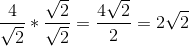# ISEE Upper Level Quantitative : How to find the volume of a cube

## Example Questions

### Example Question #312 : Geometry

Which is the greater quantity?

(a) The volume of a cube with surface areainches

(b) The volume of a cube with diagonalinches

(b) is greater.

(a) is greater.

(a) and (b) are equal.

It is impossible to tell from the information given.

(b) is greater.

Explanation:

The cube with the greater sidelength has the greater volume, so we need only calculate and compare sidelengths.

(a)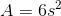, so the sidelength of the first cube can be found as follows: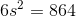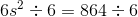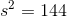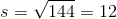inches

(b)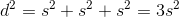by an extension of the Pythagorean Theorem, so the sidelength of the second cube can be found as follows: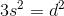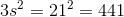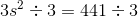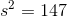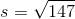Since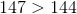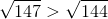. The second cube has the greater sidelength and, subsequently, the greater volume. This makes (b) greater.

### Example Question #313 : Geometry

Cube 2 has twice the sidelength of Cube 1; Cube 3 has twice the sidelength of Cube 2; Cube 4 has twice the sidelength of Cube 3.

Which is the greater quantity?

(a) The mean of the volumes of Cube 1 and Cube 4

(b) The mean of the volumes of Cube 2 and Cube 3

It cannot be determined from the information given.

(b) is greater.

(a) is greater.

(a) and (b) are equal.

(a) is greater.

Explanation:

The sidelengths of Cubes 1, 2, 3, and 4 can be given values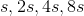, respectively.

Then the volumes of the cubes are as follows:

Cube 1: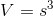Cube 2: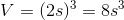Cube 3: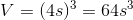Cube 4: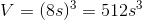In both answer choices ask for a mean, so we can determine which answer (mean) is greater simply by comparing the sums of volumes.

(a) The sum of the volumes of Cubes 1 and 4 is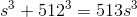.

(b) The sum of the volumes of Cubes 2 and 3 is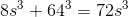.

Regardless of, the sum of the volumes of Cubes 1 and 4 is greater, and therefore, so is their mean.

### Example Question #314 : Geometry

What is the volume of a cube with side length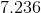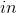? Round your answer to the nearest hundredth.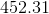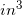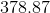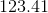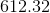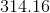Explanation:

This question is relatively straightforward. The equation for the volume of a cube is:(It is like doing the area of a square, then adding another dimension!)

Now, for our data, we merely need to "plug and chug:"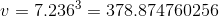### Example Question #315 : Geometry

What is the volume of a cube on which one face has a diagonal of?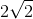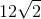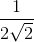Explanation:

One of the faces of the cube could be drawn like this: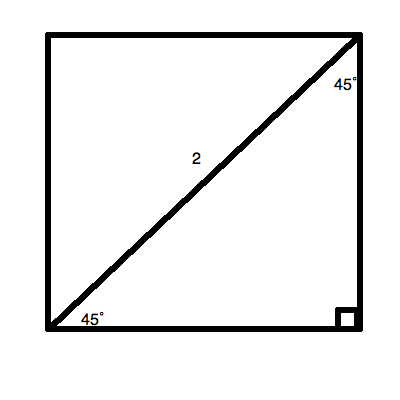Notice that this makes a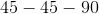triangle.

This means that we can create a proportion for the sides. On the standard triangle, the non-hypotenuse sides are both, and the hypotenuse is.  This will allow us to make the proportion: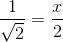Multiplying both sides by, you get: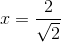Recall that the formula for the volume of a cube is:Therefore, we can compute the volume using the side found above: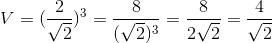Now, rationalize the denominator: